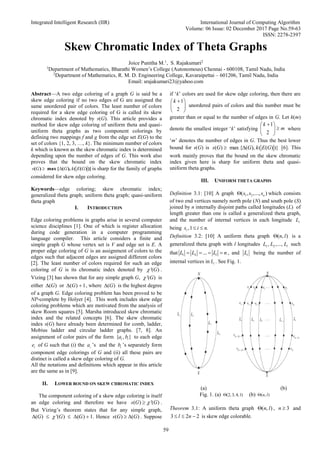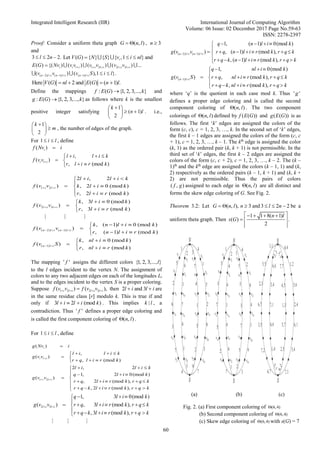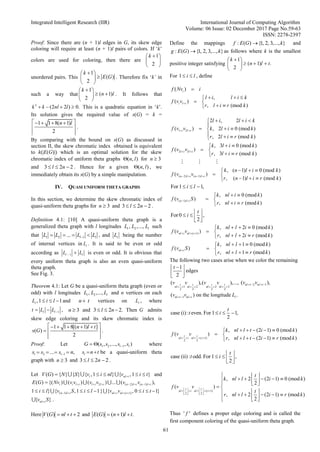Diese Präsentation wurde erfolgreich gemeldet.

# Skew Chromatic Index of Theta Graphs

Anzeige
Anzeige
Anzeige
Anzeige
Anzeige
Anzeige
Anzeige
Anzeige
Anzeige
Anzeige
AnzeigeAnzeige
×

1 von 5 Anzeige

# Skew Chromatic Index of Theta Graphs

Abstract—A two edge coloring of a graph G is said be a skew edge coloring if no two edges of G are assigned the same unordered pair of colors. The least number of colors required for a skew edge coloring of G is called its skew chromatic index denoted by s(G). This article provides a method for skew edge coloring of uniform theta and quasi-uniform theta graphs as two component colorings by defining two mappings f and g from the edge set E(G) to the set of colors {1, 2, 3, …, k}. The minimum number of colors k which is known as the skew chromatic index is determined depending upon the number of edges of G. This work also proves that the bound on the skew chromatic index is sharp for the family of graphs considered for skew edge coloring.

Abstract—A two edge coloring of a graph G is said be a skew edge coloring if no two edges of G are assigned the same unordered pair of colors. The least number of colors required for a skew edge coloring of G is called its skew chromatic index denoted by s(G). This article provides a method for skew edge coloring of uniform theta and quasi-uniform theta graphs as two component colorings by defining two mappings f and g from the edge set E(G) to the set of colors {1, 2, 3, …, k}. The minimum number of colors k which is known as the skew chromatic index is determined depending upon the number of edges of G. This work also proves that the bound on the skew chromatic index is sharp for the family of graphs considered for skew edge coloring.

Anzeige
Anzeige